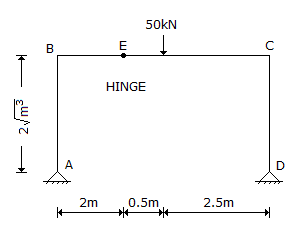# Civil Engineering - UPSC Civil Service Exam Questions

26.

The ture bearing of a line is 34° 20' 40"and the magnetic declination at the place of observation is 2° 00' 20" W on the date of observation. The magnetic bearing of the line is

 A. 36° 21' 00" B. 34° 20' 20" C. 32° 20' 20" D. 32° 00' 20"

Explanation:

No answer description available for this question. Let us discuss.

27.

What is the inclination of resultant reactions at A with the vertical for the frame shown in the given figure ?A. 60° B. 40° C. 30° D. 50°

Explanation:

No answer description available for this question. Let us discuss.

28.

Which one of the following, measures the stiffness of a track ?

 A. Tractive effort B. Tractive resistance C. Track modulus D. Load capacity

Explanation:

No answer description available for this question. Let us discuss.

29.

Consider the following statements :
1. About 25% of alumina is brick earth imparts the plasticity necessary for moulding bricks into required shape.
2. Iron pyrite present in brick earth preserves the form of the bricks at high temperatures.
3. Presence of weeds in brick earth makes the bricks unsound.
Which of these statements are correct ?

 A. 1 and 2 B. 1 and 3 C. 2 and 3 D. 1, 2 and 3

Explanation:

No answer description available for this question. Let us discuss.

30.

In the cross-section of a rectangular beam, what is the ratio of the average shear stress to the maximum shear stress ?

 A. 3/2 B. 2/3 C. 4/3 D. 3/4